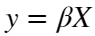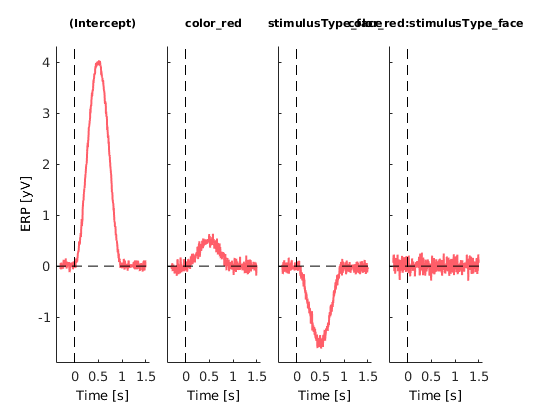init_unfold
Starting unfold toolbox.
Adding subfolders and other toolboxes to path...
Done.

# Introduction

This basic tutorial starts with generating some EEG data with overlap. For the sake of having a backstory, we will simulate data of an experiment where we have two types of stimuli (face or car) with two types of colors (red and green) embedded in pink noise. Subjects are asked to count the number of objects irregardless of color.
We are interested what the influences of the stimulus-type and color is.
This tutorial only shows the minimally necessary functions. Have a look at the second tutorial for more extensive explanations and plotting functions.
% We will first simulate data based on the car/face, green/red design.
EEG = tutorial_simulate_data('2x2')
eeg_checkset note: upper time limit (xmax) adjusted so (xmax-xmin)*srate+1 = number of frames
EEG = struct with fields:
setname: ''
filename: ''
filepath: ''
subject: ''
group: ''
condition: ''
session: []
nbchan: 1
trials: 1
pnts: 120000
srate: 200
xmin: 0
xmax: 599.9950
times: [1×120000 double]
data: [1×120000 single]
icaact: []
icawinv: []
icasphere: []
icaweights: []
icachansind: []
chanlocs: []
urchanlocs: []
chaninfo: [1×1 struct]
ref: 'common'
event: [413×1 struct]
urevent: []
eventdescription: {'' '' '' '' ''}
epoch: []
epochdescription: {}
reject: [1×1 struct]
stats: [1×1 struct]
specdata: []
specicaact: []
splinefile: ''
icasplinefile: ''
dipfit: []
history: '↵EEG.etc.eeglabvers = '14.1.1'; % this tracks which version of EEGLAB is being used, you may ignore it'
saved: 'no'
etc: [1×1 struct]
sim: [1×1 struct]

# Defining the 2x2 factorial design

A 2x2 anova is equal to a linear model with two categorical predictors and the interaction. We adopt the widely popular 'formula'-style of model definition. In this case 'y ~ A + B + A:B' (equivalent: 'y ~ A*B') represents the whole model. A and B are our main effects, A:B the interaction. We should specify that we have two categorical factors and we have to specify on which event(s) we want to epoch our data and evaluate the model.
cfgDesign = [];
cfgDesign.eventtypes = {'fixation'};
cfgDesign.formula = 'y ~ 1+ cat(stimulusType)*cat(color)';
EEG = uf_designmat(EEG,cfgDesign);
Modeling 413 event(s) of [fixation] using formula: y~1+stimulusType*color

# Timeshift

In order to compensate for the inevitable linear overlap between ERPs, we want to use timeshifting / prepare the designmatrix for the deconvolution step. In this step we generate for each column of the designmatrix timeshifted (by t = 1, t=2, t=3 ...) and append them to the designmatrix.
cfgTimeexpand = [];
cfgTimeexpand.timelimits = [-.3,1.5];
EEG = uf_timeexpandDesignmat(EEG,cfgTimeexpand);
uf_timeexpandDesignmat(): Timeexpanding the designmatrix...
...done

# Fit the model

We solve the linear equation:(y=data, X= Designmatrix) for β at each point in time. These betas reflect our parameter estimates.
EEG= uf_glmfit(EEG);
uf_glmfit(): Fitting deconvolution model...solving the equation system

solving electrode 1 (of 1 electrodes in total)... 35 iterations, took 0.1s
LMfit finished

# Plot the results

We choose a single electrode and plot all effects.
ufresult= uf_condense(EEG);
cfg = [];
cfg.channel = 1;
ax = uf_plotParam(ufresult,cfg);
plotting all parameters
ordering given as valuesOne can clearly see that we recover the intercept and the simulated effects. The intercept represents the response when both stimulusType = 'car' and color = 'green'. The second column is the component one needs to add to the intercept to gain the ERP for the color 'red'. The third column equally represents the difference (i.e. what to add) of face to car. The fourth column represents the interaction. Because we did not simulate an interaction, this ERP is flat. Note that with effects-coding the results would look quite different. Have a look at tutorial 2 where we go into more depth.